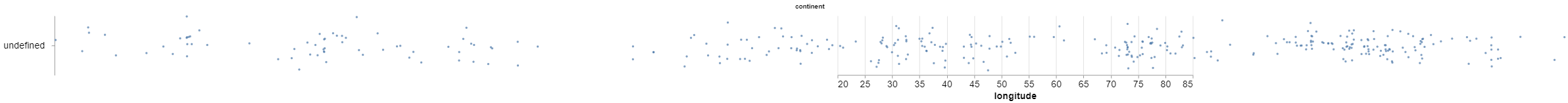Related Articles
Horizontal Stripplot with Jitter using Altair in Python
• Difficulty Level : Hard
• Last Updated : 13 Jan, 2021

Prerequisites: Altair

Altair is a statistical data visualization library in python which is based on Vega and Vega-Lite visualization grammars. A Stripplot is used for graphical data analysis.  It is a simple plot of response values in a sorted order along a single axis. The strip plot consists of 2 distinct axes (X, Y). The strip plots provide an alternative for the histogram and other density-based plots and are often used with small datasets.

A simple strip plot is used for plotting the data as points, which may not be very useful to us. To make the simple stripplot more cultivate we add random jitter. Jitter in simple words is adding a small amount of variability(horizontal or vertical) to the data to ensure all data points are visible. In this article we will discuss how it will be achieved horizontally.

### Approach

• Import module
• Now, to create the horizontal stripplot we will interchange our x-axis and y-axis.
• Define jitter for the plot
• Plot the graph
• Display plot

### Function Used

calculate_transform() allows the user to define new fields in the dataset which are calculated from other fields using an expression.

Syntax:

`calculate_transform(<some_expression>)`

Program:

## Python3

 `import` `altair as alt``import` `pandas as pd``import` `numpy as np`` ` ` ` `data_url ``=` `'https://raw.githubusercontent.com/curran/data/gh-pages/geonames/cities1000000.csv'``df ``=` `pd.read_csv(data_url)``df.head()`` ` ` ` `horizontal_stripplot ``=` `alt.Chart(df, width``=``600``, height``=``100``).mark_circle(size``=``12``).encode(``    ``y``=``alt.Y(``        ``'jitter:Q'``,``        ``title``=``None``,``        ``axis``=``alt.Axis(values``=``[``0``], ticks``=``True``, grid``=``False``, labels``=``False``),``        ``scale``=``alt.Scale(),``    ``),``    ``x``=``alt.X(``'longitude:Q'``, scale``=``alt.Scale(domain``=``(``20``, ``85``))),``    ``color``=``alt.Color(``'continent:N'``, legend``=``None``),``    ``row``=``alt.Row(``        ``'continent:N'``,``        ``header``=``alt.Header(``            ``labelAngle``=``0``,``            ``labelFontSize``=``16``,``            ``titleOrient``=``'top'``,``            ``labelOrient``=``'left'``,``            ``labelAlign``=``'left'``,``        ``),``    ``),``).transform_calculate(``    ``# Generate Gaussian jitter with a Box-Muller transform``    ``jitter``=``'sqrt(-2*log(random()))*cos(2*PI*random())'``).configure_facet(``    ``spacing``=``0``).configure_view(``    ``stroke``=``None``).configure_axis(``    ``labelFontSize``=``16``,``    ``titleFontSize``=``16``)`` ` ` ` `horizontal_stripplot`

Output:Attention geek! Strengthen your foundations with the Python Programming Foundation Course and learn the basics.

To begin with, your interview preparations Enhance your Data Structures concepts with the Python DS Course. And to begin with your Machine Learning Journey, join the Machine Learning – Basic Level Course

My Personal Notes arrow_drop_up